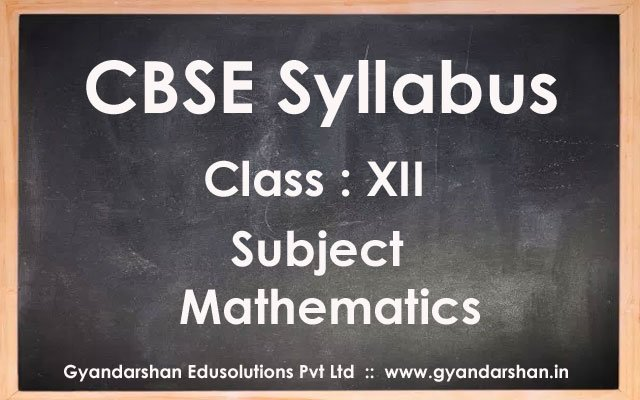Home CBSE Syllabus Mathematics Class XII Syllabus

# Mathematics Class XII Syllabus

286
0### Exam Structure

 Unit Topic Marks I. Relations and Functions 10 II. Algebra 13 III. Calculus 44 IV. Vectors and 3-D Geometry 17 V. Linear Programming 06 VI. Probability 10 Total 100

### Unit I: Relations and Functions

1. Relations and Functions

Types of relations: reflexive, symmetric, transitive and equivalence relations. One to one and onto functions, composite functions, inverse of a function. Binary operations.

2. Inverse Trigonometric Functions

Definition, range, domain, principal value branch. Graphs of inverse trigonometric functions. Elementary properties of inverse trigonometric functions.

### Unit II: Algebra

1. Matrices

Concept, notation, order, equality, types of matrices, zero and identity matrix, transpose of a matrix, symmetric and skew symmetric matrices. Operation on matrices: Addition and multiplication and multiplication with a scalar. Simple properties of addition, multiplication and scalar multiplication. Noncommutativity of multiplication of matrices and existence of non-zero matrices whose product is the zero matrix (restrict to square matrices of order 2).Concept of elementary row and column operations. Invertible matrices and proof of the uniqueness of inverse, if it exists; (Here all matrices will have real entries).

2. Determinants

Determinant of a square matrix (up to 3 x 3 matrices), properties of determinants, minors, co-factors and applications of determinants in finding the area of a triangle. Adjoint and inverse of a square matrix. Consistency, inconsistency and number of solutions of system of linear equations by examples, solving system of linear equations in two or three variables (having unique solution) using inverse of a matrix.

### Unit III: Calculus

1. Continuity and Differentiability

Continuity and differentiability, derivative of composite functions, chain rule, derivatives of inverse trigonometric functions, derivative of implicit functions. Concept of exponential and logarithmic functions.

Derivatives of logarithmic and exponential functions. Logarithmic differentiation, derivative of functions expressed in parametric forms. Second order derivatives. Rolle’s and Lagrange’s Mean Value Theorems (without proof) and their geometric interpretation.

2. Applications of Derivatives

Applications of derivatives: rate of change of bodies, increasing/decreasing functions, tangents and normals, use of derivatives in approximation, maxima and minima (first derivative test motivated geometrically and second derivative test given as a provable tool). Simple problems (that illustrate basic principles and understanding of the subject as well as real-life situations).

3. Integrals

Integration as inverse process of differentiation.Integration of a variety of functions by substitution, by partial fractions and by parts, Evaluation of simple integrals of the following types and problems based on them.Definite integrals as a limit of a sum, Fundamental Theorem of Calculus (without proof). Basic propertiesof definite integrals and evaluation of definite integrals.

4. Applications of the Integrals

Applications in finding the area under simple curves, especially lines, circles/parabolas/ellipses (in standard form only), Area between any of the two above said curves (the region should be clearly identifiable).

5. Differential Equations

Definition, order and degree, general and particular solutions of a differential equation.Formation of differential equation whose general solution is given.Solution of differential equations by method of separation of variables solutions of homogeneous differential equations of first order and first degree. Solutions of linear differential equation of the type:

dy/dx + py = q, where p and q are functions of x or constants.

dx/dy + px = q, where p and q are functions of y or constants.

### Unit IV: Vectors and Three-Dimensional Geometry

1. Vectors

Vectors and scalars, magnitude and direction of a vector.Direction cosines and direction ratios of a vector. Types of vectors (equal, unit, zero, parallel and collinear vectors), position vector of a point, negative of a vector, components of a vector, addition of vectors, multiplication of a vector by a scalar, position vector of a point dividing a line segment in a given ratio. Definition, Geometrical Interpretation, properties and application of scalar (dot) product of vectors, vector (cross) product of vectors, scalar triple product of vectors.

2. Three – dimensional Geometry

Direction cosines and direction ratios of a line joining two points.Cartesian equation and vector equation of a line, coplanar and skew lines, shortest distance between two lines.Cartesian and vector equation of a plane.Angle between (i) two lines, (ii) two planes, (iii) a line and a plane.Distance of a point from a plane.

### Unit V: Linear Programming

1. Linear Programming

Introduction, related terminology such as constraints, objective function, optimization, different types of linear programming (L.P.) problems, mathematical formulation of L.P. problems, graphical method of solution for problems in two variables, feasible and infeasible regions (bounded and unbounded), feasible and infeasible solutions, optimal feasible solutions (up to three non-trivial constraints).

### Unit VI: Probability

1. Probability

Conditional probability, multiplication theorem on probability. independent events, total probability, Baye’s theorem, Random variable and its probability distribution, mean and variance of random variable. Repeated independent (Bernoulli) trials and Binomial distribution.

Previous articleLegal Studies Class XII Syllabus
Next articlePhysics Class XII Syllabus